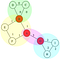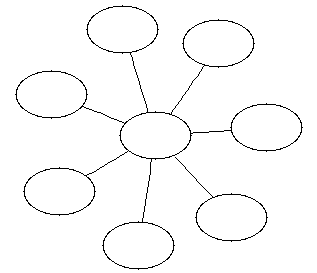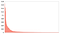# Measuring Node Centrality in Lightning Network

When people say “the Lightning Network (LN) is centralised”, we should respond by publishing metrics of the LN graph that can help refute such claims. We can start by measuring well known graph metrics like centrality, central point dominance and number of articulation points. This will help inform the on-going development of LN.

In this post I focus on above three metrics. Centrality of a node in a graph is a measure of how often shortest paths between any two nodes in the graph will include this node. Central Point Dominance measures the highest centrality of the graph. Articulation points are nodes whose failure will partition the network. These metrics enable us to answer questions about centralisation of LN.

I analysed the LN graph as obtained by describegraph command on August 24th 2018 and calculated the centrality of all nodes and found that the graph’s central point dominance was quite low (0.1668). However failure of certain articulation point nodes could partition the network — the latter is something that the community can improve quite easily by adjusting the open channels between nodes.

In the remainder of the article, I first provide a brief background to routing in Lightning Network, followed by a short overview of the graph algorithms I used in this analysis. I present the results obtained, and finally motivate future work to provide better metrics for LN.

## Background

The lightning network routes payment requests using a graph of nodes where the edges are the channels between the nodes. The route to send the payment is determined by the node sending the payment based on its view of the Lightning Network. If a payment via a route fails, the sending node tries a different route.

This raises two questions

1. Will there be a relatively small number of important nodes through which a significant majority of payments have to be routed through?
2. How easily will Lightning Network be partitioned in case of node failures, especially nodes with many open channels or like the nodes in point 1 above?

I try to answer these questions using well understood metrics of centrality, central point dominance and articulation points. I provide a brief introduction to these concepts and then present the results I obtained on Aug 24th 2018 for Lightning Network mainnet.

# Graph Algorithms — quick recap

A graph is made of nodes and edges that connect those nodes. Edges can be either directed or undirected. Nodes have paths to each other, where a path is the set of edges to be traversed to get from one node to the other. A connected graph is one where all nodes have paths to all other nodes in the graph.

For this post, I treat the LN graph as an undirected graph and all the algorithms used here are applicable only to undirected graphs. I leave the determining of similar metrics for directed graphs as future work.

## Biconnected Components and Articulation Points

A connected graph is biconnected if removing any single node results in the graph getting disconnected, i.e. have two or more disconnected components. The node that is removed to disconnect the graph is called an articulation point of the graph. Each of the disconnected graphs are called biconnected components. A graph can be disconnected into a number of biconnected components and therefore have a number of articulation points.

The image below shows an example biconnected graph, that has four biconnected components identified by labels 0,1,2,3 on the edges. The graph has three articulation points, identified by node names A, B and G.An example of a biconnected graph. Nodes B, A and G are the articulation points for the graph. (Image source: boost graph library documentation).

Tracking the number of articulation points and the sizes of the biconnected components will help the community be aware of the robustness of the Lightning Network as it grows.

We want LN to have as few articulation points as possible. However, in LN there are a lot of leaf nodes, i.e. nodes that have a single channel open to another node. To avoid leaf nodes skewing the results, I count only those articulation points that connect to at least two biconnected components with five nodes or more.

## Centrality

Freeman’s seminal paper published in 1977, presented an approach to determine the centrality of a node, n, in the graph as the number of all pairs shortest paths in the graph that include the node n. The paper then defines relative centrality for a node, so that centrality values from different graphs can be compared and are not affected by the size of the different graphs.

Central point dominance measures the maximum centrality of a node in a graph. This value ranges from 0 to 1, where it is 0 when there is no node such that all shortest paths have to pass through it. On the other hand, a value of 1 means all routes have to pass through that node.

For example, the figure below shows an example of a graph with central point dominance of 1. We want the central point dominance of the LN graph to be as close to 0 as possible, i.e. lower is good.All shortest paths between all nodes pass through a single node. Central point dominance = 1.

# Rephrasing the Questions

The questions from earlier in this post can be rephrased in terms of the metrics described above.

1. What is the central point dominance of the Lightning Network? By calculating this metric, a measure of how often a payment will pass through one or more important nodes is obtained. Ideally, for LN, this number should be as low as possible.
2. Are there any articulation points in the Lightning Network? If so, how many and how large are the biconnected components? This helps us understand how vulnerable the network is to partitioning if one or more nodes fail. Again, we want the network to have as few articulation points as possible.

An additional observation is whether there is an overlap between articulation points and nodes with the highest centrality values. This helps us understand how critical certain nodes are for keeping LN connected and routing payments successfully between nodes.

# Results

I processed the output of the describegraph command from LND and use Boost Graph Library to compute the metrics described above. When I got the graph from lncli I was using version 0.4.2 on commit 625b210.

The source code for processing the output of describegraph and run the BGL algorithms is available in two repos at https://github.com/kulpreet/lightning-network-graphxml and https://github.com/kulpreet/lightning-network-graph-analysis.

To find the centrality, biconnected components and the articulation points of the network I used the following algorithms available in BGL:

1. Lindon C. Freeman, “A Set of Measures of Centrality Based on Betweenness”, Sociometry 40, pp. 35–41, 1977 for the centrality calculations and
2. Algorithms described in Robert E. Tarjan, “Depth first search and linear graph algorithms” SIAM Journal on Computing, 1(2):146–160, 1972 for calculating the articulation points and the biconnected components.

I used the output of describegraph on 24nd August 2018 10:17:06 UTC and got the following observations. My lnd node could see 2162 nodes.

## Central Point Dominance

LN’s central point dominance was 0.166874 and the node with the highest centrality is “tady je slushovo”.

I want to see how the central point dominance evolves. Over the last week, I have seen this number fall from 0.19 to the current 0.1668. Future work would be to chart the daily central point dominance of LN.

Apart from the central point dominance, I also computed the centrality of each node. To help us see how things are evolving, here are the top 20 nodes with the highest centrality values.

The following chart shows the top 200 nodes sorted by their centrality. There is an expected long tail of centrality, even if the central point dominance is low. I speculate that the community expects the central point dominance to reduce further as the network grows. Upon this happening, nodes will find multiple alternate routes in the presence of node failures.Centrality of 200 nodes, showing the long tail

## Articulation Points

There are more than two hundred articulation points in LN as of August 24th 2018. However, most of these articulation points break up the graph into biconnected components with a single node. To get meaningful results, I filtered the list of all articulation points to only show articulation points that connect at least two components of at least five nodes each. There are 20 such articulation points satisfying this criteria.

Note how the nodes rank when looking at the centrality vs when looking at the articulation points. For example, the ACINQ node is connecting the most number of biconnected components, but its centrality is not the highest. In contrast mainnet.lightningconductor.net is in the list of top articulation points but does not make it to the list of top 20 nodes by centrality.

# Conclusions

So here are the answers to the questions I raised earlier in the post.

1. The centrality of the Lightning Network was 0.1668 on August 24th 2018. There are other nodes with comparable centrality. This tells us is that there should be a number of ways to route a payment request between nodes.
2. There are over two hundred articulation points in LN. However, most are simply connecting biconnected components with a single node. Having said that, there are 20 nodes whose failure will partition LN. There is a lot more work needed here to answer how robust the network is over time. Future work will investigate this question and hopefully make suggested improvements to LN to make it more resilient to DOS attacks.
3. There is an overlap between articulation points and nodes with high centrality. There are some nodes that are high in centrality, implying a lot of payments could be routed through them, and they are also high in the list of articulation points.

# Future Work

There’s a lot that can be done to improve the results above. In this post I just make a first attempt at calculating metrics of the Lightning Network. Here are some possible directions:

1. Treat the lightning network graph as a directed graph instead of the simplified undirected graph approach I have taken here.
2. Use total capacity and capacity in each direction while finding centrality. This is a challenge, as the well known algorithms on centrality are only for undirected graphs.
3. Exclude leaf nodes from the lighting graph before finding centrality so that we can look at how the non edge nodes can be threatened with a network partition.
4. Start looking at how to compute maximum flow through LN given the unique characteristics of the network.
5. Plot the central point dominance and number of articulation points on a daily basis, so that the community can look at the centrality of the network at a quick glance.
6. Determine articulation points using a weighted directed graph with weights computed from channel fees and capacity values.

Written by

## Kulpreet Singh

#### “In 20 years there will either be very large transaction volume or no volume” — Satoshi

Welcome to a place where words matter. On Medium, smart voices and original ideas take center stage - with no ads in sight. Watch
Follow all the topics you care about, and we’ll deliver the best stories for you to your homepage and inbox. Explore
Get unlimited access to the best stories on Medium — and support writers while you’re at it. Just \$5/month. Upgrade